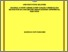# Diagonal R-point variable step variable order block method for solving second order ordinary differential equations

## Citation

Zainuddin, Nooraini (2016) Diagonal R-point variable step variable order block method for solving second order ordinary differential equations. Doctoral thesis, Universiti Putra Malaysia.

## Abstract

This thesis focuses on solving the initial value problems of stiff second order Ordinary Differential Equations (ODEs) directly by methods of 2-point Diagonal Block Backward Differentiation Formula (2DBBDF) and 3-point Diagonal Block Backward Differentiation Formula (3DBBDF). The 2DBBDF and 3DBBDF give two and three approximated solutions respectively for each integration step. The coefficients of these methods are derived by utilizing the error constants of the linear difference operators obtained from the general form of each method. The convergence and stability properties of the 2DBBDF and 3DBBDF methods are also discussed in details. The proposed 2DBBDF and 3DBBDF methods are implemented with fixed step size in order to justify the numerical efficiency of the proposed methods. Subsequently, the computation of variable step size of 2DBBDF and 3DBBDF methods of order two, three and four are presented and finally they are implemented in variable step variable order scheme. The detailed algorithms on the selection of step sizes and orders are discussed. Conclusively, numerical results obtained while comparing the proposed methods with the existing variable step methods show the efficiency in reducing the number of function evaluations as well as the number of total steps. Further implementation of the 2DBBDF and 3DBBDF methods in variable step variable order scheme displays comparable results with other variable step variable order methods. These findings conclude that the proposed methods can serve as an alternative solver for solving stiff second order ODEs directly.Preview
Text
FS 2016 67 IR.pdfView Item